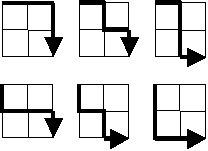### The Problem

Starting in the top left corner of a 2×2 grid, and only being able to move to the right and down, there are exactly 6 routes to the bottom right corner.How many such routes are there through a 20×20 grid?

### A Note Before We Begin

I have seen several, more clever solutions to this problem. I've seen one that starts at the bottom and works up, and another that manages to reduce the problem to a simple equation (the math of which is beyond me). So this isn't the best solution by any stretch, but it's the one that works for me.

### The Solution

This is another problem where pure bute force doesn't do the trick. But let's look at that approach first.

``````[<Literal>]
let GridSize = 20

let countRoutesTo sourceX sourceY destX destY =

let rec count sx sy =
if sx = destX && sy = destY then
1L
elif sx < GridSize && sy < GridSize then
(count (sx+1) sy) + (count sx (sy+1))
elif sx < GridSize then
(count (sx+1) sy)
else
(count sx (sy+1))

let result = count 0 0
result

#time
countRoutesTo 0 0 GridSize GridSize
#time
``````

The internal `count` function is a recursive function that computes all of the routes to `destX,destY`. It works like this:

• If we've hit the bottom right corner, the result is always 1 since we had to have made it there from one of the points adjacent to the bottom corner.
• If we're somewhere in the interior of the grid, we return `count` for a move right plus a `count` for a move down.
• If we're at the bottom edge, we return `count` for a move right.
• Otherwise, we must be at the right edge, so we return `count` for a move down.

The problem with this function is that it takes way too long to execute. I stopped it running after 20 minutes. I know it works in principle because if I reduce `GridSize` to 10, it executes in under a second. The problem is that the number of calls to `count` is a factorial of the GridSize. In fact, you can get the answer to the problem by computing (2n)!/n!2, but I didn't know that when I was writing this :)

My (ahem!) genius solution to the problem was to cache the answers for the lower right 100 squares of the grid, and then apply my brute force algorithm. If the algorithm hits a cached position, it doesn't have to do any more work and just returns the value it's currently computed plus the value from the cache.

This algorithm finds the answer in 22 seconds on my machine.

``````let countRoutesToCached sourceX sourceY destX destY =

let cachePos = GridSize / 2
let cache = System.Collections.Generic.Dictionary<int*int,int64>()

let rec count sx sy =
match cache.TryGetValue((sx,sy)) with
| true,v -> v
| _ ->
if sx = destX && sy = destY then
1L
elif sx < GridSize && sy < GridSize then
(count (sx+1) sy) + (count sx (sy+1))
elif sx < GridSize then
(count (sx+1) sy)
else
(count sx (sy+1))

for x in cachePos..GridSize do
for y in cachePos..GridSize do
cache.Add((x,y),(count x y)) |> ignore

let result = count 0 0
result

#time
countRoutesToCached 0 0 GridSize GridSize
#time
``````
Other Posts in This Series